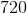# SAT II Math II : Radians and the Unit Circle

## Example Questions

### Example Question #1 : Radians And The Unit Circle

The degree anglecan be expressed as what in radians?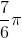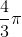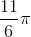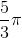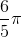Explanation:

In order to convert degrees to radians, we will need to know the conversion factor.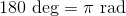Set up a dimensional analysis to solve.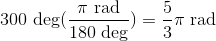The answer is:### Example Question #2 : Radians And The Unit Circle

Which of the following angles belong in the fourth quadrant?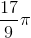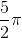Explanation:

The fourth quadrant is in the positive x-axis and negative y-axis.

The angle ranges are: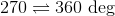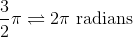The only possible answer is:### Example Question #3 : Radians And The Unit Circle

What degree measure is equivalent to?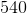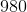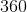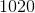Explanation:

Every pi radians is equal to 180 degrees.

Replace the pi term with 180 degrees and multiply.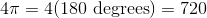The answer is: